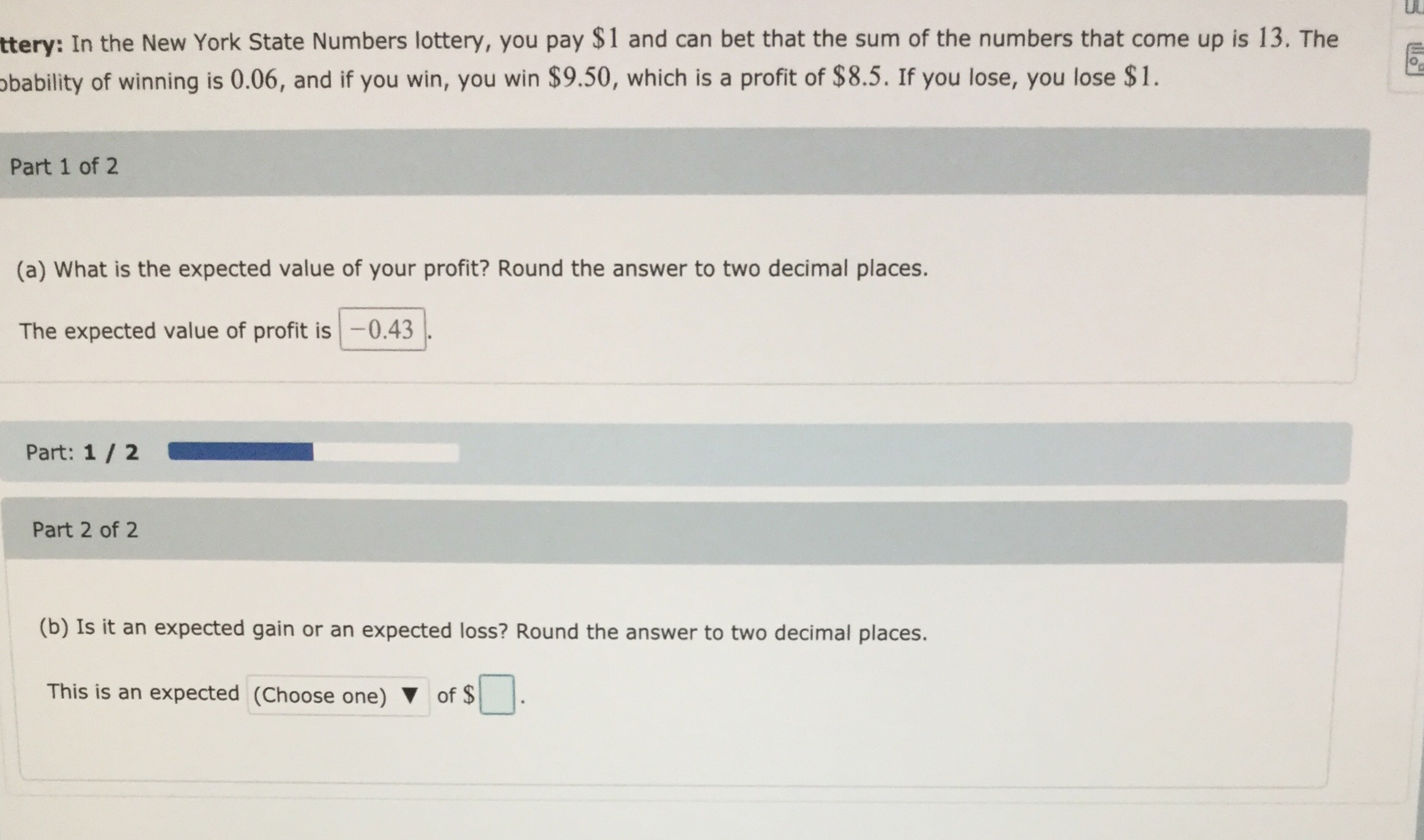# UCttery: In the New York State Numbers lottery, you pay \$1 and can bet that the sum of the numbers that come up is 13. Thebability of winning is 0.06, and if you win, you win \$9.50, which is a profit of \$ 8.5. If you lose, you lose \$1Part 1 of 2(a) What is the expected value of your profit? Round the answer to two decimal places.The expected value of profit is-0.43Part: 1/ 2Part 2 of 2(b) Is it an expected gain or an expected loss? Round the answer to two decimal places.This is an expected (Choose one)of \$

Question
9 viewshelp_outlineImage TranscriptioncloseUC ttery: In the New York State Numbers lottery, you pay \$1 and can bet that the sum of the numbers that come up is 13. The bability of winning is 0.06, and if you win, you win \$9.50, which is a profit of \$ 8.5. If you lose, you lose \$1 Part 1 of 2 (a) What is the expected value of your profit? Round the answer to two decimal places. The expected value of profit is-0.43 Part: 1/ 2 Part 2 of 2 (b) Is it an expected gain or an expected loss? Round the answer to two decimal places. This is an expected (Choose one) of \$ fullscreen
check_circle

Step 1

(a)

The expected value of profit is obtained below:

There is 0.06 chance of profit is \$8.50, there is a 0.94 chance of loss of \$1.

The Expected value of formula is,

Step 2

The expected value o...

### Want to see the full answer?

See Solution

#### Want to see this answer and more?

Solutions are written by subject experts who are available 24/7. Questions are typically answered within 1 hour.*

See Solution
*Response times may vary by subject and question.
Tagged in

### Other